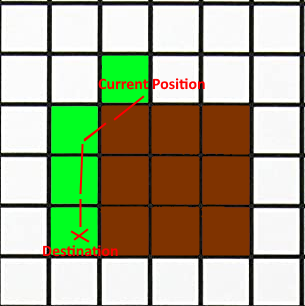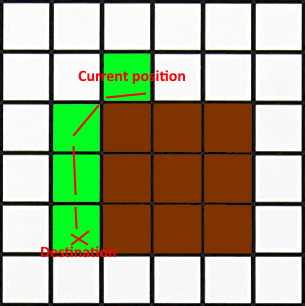# How to get all nodes from current position to next position

When CalculateNextPosition is called from AILerp it gets the position through a tangent, this position can be a node not attached to the current node, so how can I get all the nodes I would go through to this new position?
and is taking into account the penalties of all these nodes through which it would pass?

Hi

I don’t quite understand what you want to do. Am I correct in that you want to get the list of all nodes (including the current one) that the agent will traverse until the end of the path?

I need to analyze all the nodes through which the agent passes, in order to decide whether to stop or continue, so I have noticed that sometimes when calculating the next position with the CalculateNextPosition method, it obtains a position to a non-adjacent node (due to interpolation or elapsed time while calculating the path, etc), then because of these jumps, I need to know all the nodes it would pass through from the current position to the position calculated by CalculateNextPosition (Nodes from current position to nextposition).

Hi

The CalculateNextPosition tracks the agent’s position on a polyline from the start of the path to the end of the path. It increments the distance along this polyline by the agent’s speed and returns the new position.

If you are subclassing (or changing) the AILerp script you could do this to predict the outcome of CalculateNextPosition:

``````var startNode = interpolator.segmentIndex;
var originalDist = interpolator.distance;
// Calculate the next position, this will modify the interpolator
var next = CalculateNextPosition(out Vector3 direction, deltaTime);
var endNode = interpolator.segmentIndex;
// Reset the interpolator from the backup
interpolator.distance = originalDist;

for (int i = startNode; i < endNode; i++) {
Debug.Log("Agent would move over node " + i + " in the path");
var node = path.path[i];
}
``````

Hello, thank you very much for the answer, it seems to work quite well but sometimes I get the following error when assigning interpolator.distance = originalDist

I also think that the variable pathSwitchInterpolationTime = originalPathSwitchInterpolationTime should be reset right?

Ok, I fixed the bug with interpolator.valid, I have another problem, when is it the case (startNode == endNode && path.path.Count <= startNode) or (startNode == endNode && path.path.Count > startNode) What would be the next position for both cases?

something is wrong, as sometimes it returns that all nodes have tag == 0, but when you then get the node’s tag from nextposition (AstarPath.active.GetNearest(nextPosition).node.Tag), it returns 1. I think this is due to the FunnelModifier and SimpleSmoothModifier modifiers, since I am allowing tag 1 in the seeker, is that right? in such a case how could I make the modifiers not get positions with tag = 1?

Hi

If you use the SimpleSmoothModifier the path may very well cross into other nodes that were not on the original path. The funnel modifier is a bit more conservative, but the path will go right on the edge between two nodes. So depending on floating point errors you may get one or the other.

I would recommend that you debug when this happens for you using `Debug.DrawRay` (and maybe `Debug.Break()`).

I’m finally using the RaycastModifier with the Use graph Raycasting option enabled, and when looping through all nodes from the start node to the next node, I see that none match AstarPath.active.GetNearest(nextPosition).node.
What is this about? I need to check exactly all the nodes the agent walks through and this doesn’t seem to work correctly, AstarPath.active.GetNearest(nextPosition).node should be contain in path.path

Code example:

``````var startNode = interpolator.segmentIndex;
var originalDist = interpolator.distance;
// Calculate the next position, this will modify the interpolator
var next = CalculateNextPosition(out Vector3 direction, deltaTime);
var endNode = interpolator.segmentIndex;
// Reset the interpolator from the backup
interpolator.distance = originalDist;
var vNodeNextPosition = AstarPath.active.GetNearest(nextPosition).node:

for (int i = startNode; i < endNode; i++) {
var node = path.path[i];
if(vNodeNextPosition.nodeIndex == node.nodeIndex)
Debug.LogError("nodeNextPosition In Array");
}
``````

Hi

`path.path` will always contain the nodes that the pathfinding system returned as the shortest path. No modifiers will modify it.

If you want to use the raycast modifier you can calculate a new list of all nodes it traverses by using a Linecast and passing the `trace` parameter. The list passed to `trace` will be filled with all nodes that the linecast traverses.
See GridGraph - A* Pathfinding Project

using linecast from the current position of the agent to the next position (red line), I get 2 nodes for which, the first node fetched does not belong to path.pat but the final one does, so I would like to know if nextposition always calculates the position of a node contained in path.path. If not (which I think is the case…) how can I correct the next position to fit the closest node contained in path.path?
As you can see the nodes of the computed path (blue line) are the nodes the agent moves through, but I need to get the start node and end node from path.path (with RaycastModifier I can’t get these indices (startnode endnode) like you mentioned above, they always return 0)

``````            var gg = AstarPath.active.data.gridGraph;
List<GraphNode> vList = new List<GraphNode>();
gg.Linecast(tr.position, nextPosition, out GraphHitInfo v, vLista);
vNodeAux = AstarPath.active.GetNearest(tr.position).node;
foreach (var vNode in vList)
{
Debug.DrawLine((Vector3)vNodeAux.position, (Vector3)vNode.position, Color.red, 0.5f);
vNodeAux= vNode;
}

//Nodes path.path
vNodo = this.NodoActual;
for (int i = 0; i < path.path.Count; i++)
{
if (this.miorganismo.DEBUG)
Debug.DrawLine((Vector3)vNodo.position, (Vector3)path.path[i].position, Color.blue, 0.5f);
vNodo = path.path[i];
}
``````

Here another example:
White lines: Represents the center of all nodes in path.path (using raycastmodifier).
Green Lines: Represents the positions of all smooth nodes of path.path (Path drawn by seeker.drawgizmos).
Blue Line: Represents the current position of the agent to the position calculated by nextposition (Sometimes the position calculated by interpolation, gives a position in an invalid node that is not in the path.path list, which is the problem with all this).
Red line: represents the center of all nodes touched by the linecast from the agent’s current position to the position calculated by nextposition.

Conclusion: Nextposition can’t return a node that doesn’t contain path.path, so to avoid this, I need to interpolate to a node contained in path.path but I don’t really know how to do it…

The intorpalation should be like the light blue line in this image:

In the following way I get the StarNode of the agent, the node before NodeNextPosition and the node after NodeNextPosition (this can be the same as the previous node since NodeNextPosition can be the last node in the path). I would like to know a cleaner way to get these nodes as this code is quite dirty and unstable.

``````GraphNode vCurrentNode = AstarPath.active.GetNearest(tr.position).node;
var gg = AstarPath.active.data.gridGraph;
List<GraphNode> vTraversedNodesList= new List<GraphNode>();
//Get the list of all the nodes that I would step on from current position to nextposition
gg.Linecast(tr.position, nextPosition, out GraphHitInfo v, vTraversedNodesList);
GraphNode vStartNode = null;
GraphNode vPreviousNodeNextPosition= null;
GraphNode vNextNodeNextPosition= null;
GraphNode vNodeAux;
//get the node from the current position and the previous and next node from nextposition
for (int i = 0; i < vTraversedNodesList.Count; i++)
{
vNodeAux= vTraversedNodesList[i];
if (vStartNode== null && vNodeAux.NodeIndex == vCurrentNode .NodeIndex)
{
vStartNode= vCurrentNode l;
//vPreviousNodeNextPosition could be the same node as the initial node
vPreviousNodeNextPosition= vCurrentNode ;
}
else if (vStartNode!= null)
{
if (vNodeAux.tag != 1)
vPreviousNodeNextPosition= vNodeAux;
else
{
if (i + 1 < vTraversedNodesList.Count)
vNextNodeNextPosition= vTraversedNodesList[i];
//The next node would be the same as the previous node since nextnode is the last node of the route.
else
vNextNodeNextPosition= vPreviousNodeNextPosition[i];
break;
}
}
}

//Here you could already interpolate over vPreviousNodeNextPosition or over vNextNodeNextPosition.
``````

Knowing this, you could now interpolate nextPosition based on vPreviousNodeNextPosition or vNextNodeNextPosition.
But I don’t know exactly how I could interpolate to the most suitable node (vPreviousNodeNextPosition or vNextNodeNextPosition). This would be the solution to my problem… I just need to know how to interpolate to one of the valid nodes obtained

Is my understanding correct that you want to:

1. Simplify the path using the raycast modifier
2. When the character moves, clamp its position to the surface of the nodes in the path.path list, making sure it never moves outside those nodes?

So essentially, each frame you want to find the closest point that lies on the surface of a set of nodes?
I’m not quite sure why you’d want this, but this seems to be what you are asking?

Note that this is a different question than asking “which nodes would path.vectorPath pass through”. That question is solved using linecast, as I mentioned previously.

Yes, that’s what I need, clamping the position to the surface of the nodes of the path.path list, making sure it never moves outside of those nodes.
In addition, the recalculated position must approach the furthest destination node possible, from the current position (with the distance from the current position of the agent to nextposition, it could know the total distance to apply vDistance = Vector3.Distance(new Vector3(tr. position.x, 0, tr.position.z), new Vector3(nextPosition.x, 0, nextPosition.z));

Well, I suppose you could loop over all the nodes in path.path and check for the closest point on that node using node.ClosestPointOnNode, then clamp the agent to the node that was closest. As a bonus, you’ll also find out in a robust way which of your nodes are in (even if the agent ends up right on the border between two nodes).

Here I show an example with images so you can understand me better:
Real Case:Desired Case:Brown = invalid tag
Green = Route calculated (Path.path or path.vectorpath)
Red = Nextpositions

So to achieve this, knowing the distance traveled from the current position to the next position, apply this distance traveled on the path, in order to obtain the node and the corrected position.
How can I get this position and node? and how can I then apply this position on the interpolator so that it updates to the new destination?
Can you write some sample code please, I have been trying for weeks to solve this problem.

To achieve a similar behavior, I assigned the following in the seeker’s Star end modifier option:
Start Point Snapping = Node Connection
End Point Snapping = Node Center (Snap to node).

This seems to work fine in this is the case, you would need to do something similar, when this is the case but if this is not the case go to the original destination point so that it goes exactly to the selected destination when it can.

Is there anything you can think of to solve this case?

Hi

Yes, using the Node Connection snapping mode would work well, I think. But make sure you are using `4.3.47` or later because a bug was fixed in that version related to this feature.

I'm using 4.3.54, this fixes the problem but it's not the desired behavior, as I mentioned I need it to do this behavior only when nextposition gets an invalid node, otherwise I want to go to the original destination

I’m using 4.3.54, this fixes the problem but it’s not the desired behavior, as I mentioned I need it to do this behavior only when nextposition gets an invalid node, otherwise I want to go to the original destination.
How can I do this, I should correct the position of the interpolator when this is the case